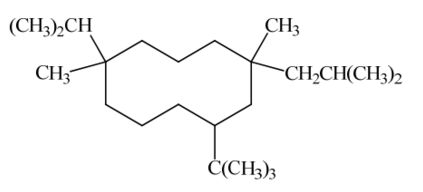# Problem: Consider the compound below to answer the following questions. (a) How many methine hydrogens are there?   (b) How many primary Cs are there?   (c) Give the molecular formula of this compound.    (d) How many quaternary Cs are there?   (e) How many secondary alkyl groups exist as substituents?

🤓 Based on our data, we think this question is relevant for Professor Hilinski's class at FSU.

###### Problem Details

Consider the compound below to answer the following questions.

(a) How many methine hydrogens are there?

(b) How many primary Cs are there?

(c) Give the molecular formula of this compound.

(d) How many quaternary Cs are there?

(e) How many secondary alkyl groups exist as substituents?What scientific concept do you need to know in order to solve this problem?

Our tutors have indicated that to solve this problem you will need to apply the Functional Groups concept. You can view video lessons to learn Functional Groups. Or if you need more Functional Groups practice, you can also practice Functional Groups practice problems.

What is the difficulty of this problem?

Our tutors rated the difficulty ofConsider the compound below to answer the following question...as medium difficulty.

How long does this problem take to solve?

Our expert Organic tutor, Jonathan took 11 minutes and 49 seconds to solve this problem. You can follow their steps in the video explanation above.

What professor is this problem relevant for?

Based on our data, we think this problem is relevant for Professor Hilinski's class at FSU.